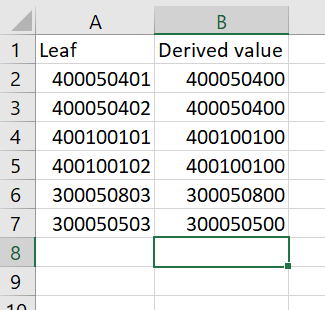# Derive a new column based on another column value

Hi,
I have data which looks something like this:The leaf column will be present by default, and I have to derive the derived value from leaf column.
For eg if leaf col value is 300050803, then the derived value would be 300050800 and so on for various values as per the excel.
What would be the best way to approach this? Couldn’t find a solution with python/ java as it isn’t allowed for now. Any other approach and help would be greatly appreciated!
Thanks!

These are numbers so I would say that with the -Math Formula- node you could do the following

1. divide / 100,
2. use the floor() function to truncate to the “largest integer below the number” the previous result
3. multiply by 100.

The equation should look like this:

`floor( \$Leaf\$ / 100) * 100`

Check before validating the “Convert to Int” option in the -Math Formula- node configuration.

Use then a -Number to String- node to convert the column to a string if needed.

Hope it helps.

Best

Ael

2 Likes

Hi @aworker , why 100? Why not divide and multiply by 10? In fact, the answer will depend on what @Saishiyam will clarify on the following question@Saishiyam , can you confirm what the derived value should be for 300050823? Is it 300050800 or 300050820?

EDIT: Basically if the answer is 300050800, then the formula `floor( \$Leaf\$ / 100) * 100` is correct.
If the answer is 300050820, however, then the formula should be `floor( \$Leaf\$ / 10) * 10`

Hi @bruno29a , if the value is 300050823, the derived column value should be 300050800.
My bad, I didn’t elaborate the question1 Like

No problem @Saishiyam and thank you for confirming.

In this case, the correct formula would be `floor( \$Leaf\$ / 100) * 100`

same result with `\$leaf\$-mod(\$leaf\$, 100)`

1 Like

@duristef

Column not found: leafBut yes, your operation with `mod()` would work too1 Like

This topic was automatically closed 90 days after the last reply. New replies are no longer allowed.